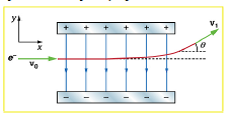# Problem: Figure 17.44 shows an electron passing between two charged metal plates that create an 100 N/C vertical electric field perpendicular to the electron's original horizontal velocity. (These can be used to change the electron's direction, such as in an oscilloscope.) The initial speed of the electron is 1.00 × 106 m/s, and the horizontal distance it travels in the uniform field is L = 10.0 cm.(a) What is its vertical deflection?(b) What is the vertical component of its final velocity?(c) At what angle  does it exit? Neglect any edge effects.

###### FREE Expert Solution

The vertical deflection of the electron is expressed as:

$\overline{){\mathbf{∆}}{\mathbf{y}}{\mathbf{=}}{{\mathbf{v}}}_{\mathbf{0}\mathbf{y}}{\mathbf{t}}{\mathbf{+}}\frac{\mathbf{1}}{\mathbf{2}}{{\mathbf{a}}}_{{\mathbf{y}}}{{\mathbf{t}}}^{{\mathbf{2}}}}$

Acceleration in the vertical direction, ay is:

$\overline{){{\mathbf{a}}}_{{\mathbf{y}}}{\mathbf{=}}\frac{\mathbf{q}\mathbf{E}}{\mathbf{m}}}$###### Problem Details

Figure 17.44 shows an electron passing between two charged metal plates that create an 100 N/C vertical electric field perpendicular to the electron's original horizontal velocity. (These can be used to change the electron's direction, such as in an oscilloscope.) The initial speed of the electron is 1.00 × 106 m/s, and the horizontal distance it travels in the uniform field is L = 10.0 cm.(a) What is its vertical deflection?
(b) What is the vertical component of its final velocity?
(c) At what angle  does it exit? Neglect any edge effects.